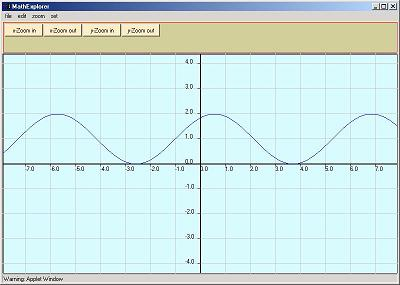# Solve Equations Graphically

The idea of solving equations graphically is explored. Many equations cannot be solved analytically hence the need for other methods of solving equations. One of these is the graphical method.

### Examples on how to solve equations graphically

Your browser is completely ignoring the <APPLET> tag!
1 - click on the button above "click here to start" and wait till a new window similar to the plot window below, pops up.

2 - click on "edit" in the main menu, and an input box, similar to the one below, pops up.

Example 1 Solve graphically the equation
(x+1)*(x+4) = -2

Note: This example is solved analytically in Tutorial on Quadratic Equations, Rational Expressions, and you can compare the answers.
How to solve the above equation graphically?
a - write your equation in standard form with one side equal to zero. The above equation is written as.
(x+1)*(x+4) + 2 = 0

b - In the main menu, click edit and a small window appears. Type in (x+1)*(x+4) + 2 and click enter. The graph corresponding to the expression entered is plotted.
c - Look for the x-intercepts of the graph. These are the points of intersection of the graph with the x-axis.
d - For this equation they should be close to -2 and -3 which are the exact solutions found in the tutorial ofTutorial on Quadratic Equations, Rational Expressions

Example 2 Solve graphically the equation
|-x + 2| = x + 1

a - write your equation in standard form with one side equal to zero. The above equation is written as.
|-x + 2| - x - 1 = 0

b - In the main menu, click edit and a small window appears. Type in abs(-x+2) - x -1 and click enter. The graph corresponding to the expression entered is plotted.

c - For this equation the solution should be close -1/2 which is the exact solution found in the tutorial ofTutorial on Quadratic Equations, Rational Expressions

Example 3 Solve graphically the equation
ln(x + 1) = x - 2
No exact analytical solution exists for this equation.

a - write your equation in standard form with one side equal to zero. The above equation is written as.
ln(x+1) - x + 2 = 0

b - In the main menu, click edit and a small window appears. Type in ln(x+1) - x + 2 and click enter. The graph corresponding to the expression entered is plotted.

c - For this equation the solutions should be close -0.94 and 3.58.

NOTE
1 - you need the multiplication symbol * between any two quantities to be multiplied. For example 2x is not allowed,
you need to type 2*x.

2 - this applet has the possibilities of zooming in and out.
2 - For a list of functions and constants that can be included in you expression click here

More references and links on how to Solve Equations, Systems of Equations and Inequalities.### input box